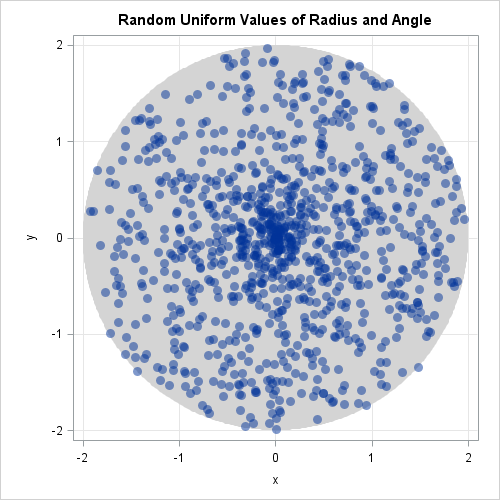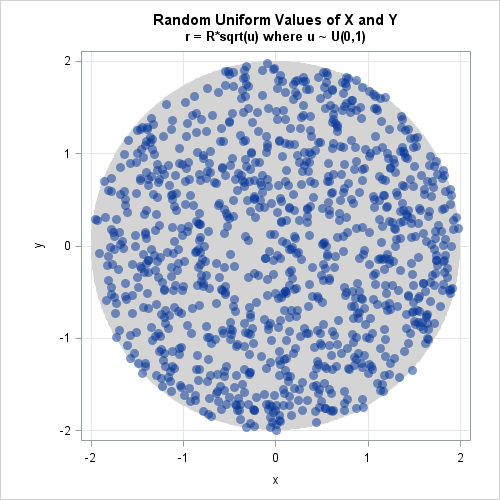It is easy to generate random points that are uniformly distributed inside a rectangle. You simply generate independent random uniform values for each coordinate. However, nonrectangular regions are more complicated. An instructive example is to simulate points uniformly inside the ball with a given radius. The two-dimensional case is to generate random points uniformly inside the planar region that contains points within a certain distance to the origin. Mathematicians call this region the two-dimensional ball or disk; others just say "inside a circle."

To generate data uniformly in any planar region, you can use the acceptance-rejection algorithm: You generate points uniformly in a bounding rectangle and then reject and discard any values that are outside the region of interest. This method always works, but it is inefficient in high dimensions (and for regions with small volumes) because most points that you generate get rejected. This article shows how to directly generate points with uniform density inside a circular region.

### How NOT to simulate data in a disk

If you are familiar with polar coordinates, you might think that this problem is simple. The function
(r, θ) → (r sin θ, r cos θ)
parameterizes a circular region in terms of radii and angles. As a first attempt, you might try to simulate uniform values of a radius and angle, and then use the polar equations to convert to Euclidean coordinates, as shown in the following SAS DATA step:

```/* This DATA step is WRONG; the points are not uniform */ data Ball1(drop=radius twopi); call streaminit(12345); radius = 2; /* use circle of radius 2 */ twopi = 2 * constant("PI"); do i = 1 to 1000; /* simulate 1000 points */ theta = twopi * rand("uniform"); /* angle is uniform */ r = radius* rand("uniform"); /* radius is uniform : THIS IS WRONG! */ x = r*cos(theta); y = r*sin(theta); output; end; run;```

Unfortunately, this simple approach is not correct. The points are uniformly distributed in the (r, θ) coordinates, but not in the (x, y) coordinates. The following scatter plot displays the (x,y) points and the circle of radius 2.It is apparent from the image that there are more points near the origin than further away from the origin. The points are not uniformly distributed.

After seeing this image, it's not hard to realize the mistake in the simulation. When we say that points should be "uniformly distributed," we mean that the probability of generating a point in any finite region is proportional to the area of that region. However, the previous program does not consider area, only angles and radii.

The area of a circle with radius r is πr2, which is proportional to the square of the radius. However, the program generates points inside a circle of radius r with a probability that is proportional to r. To be correct, the probability should be proportional to the square of r.

### How to simulate data in a disk

You can adjust the probability by changing the distribution of the r variable. Instead of sampling r uniformly, sample according to the square root of the uniform density (Devroye, 1986, p. 236). This density decreases the likelihood of points being generated near the origin and increases the probability that points will be generated near the edge of the circular region. Only one statement in the DATA step needs to change. The following SAS DATA step is the correct way to simulate points uniformly in a disk:

```/* correct way to generate points uniformly in a 2-D circular region (ball) */ data Ball(drop=radius twopi); call streaminit(12345); radius = 2; /* use circle of radius 2 */ twopi = 2 * constant("PI"); do i = 1 to 1000; /* simulate 1000 points */ theta = twopi * rand("uniform"); /* angle is uniform */ r = radius * sqrt( rand("uniform") ); /* radius proportional to sqrt(U), U~U(0,1) */ x = r*cos(theta); y = r*sin(theta); output; end; run;```The new graph of the simulation shows that the points are uniformly distributed within the circular region. You can use PROC KDE to verify that the density inside the circle is approximately constant and equal to 1/(4π), which is 1 divided by the area of the circular region.

In a future post, I will show how to generalize this example and simulate random points uniformly within a d-dimensional ball for any d ≥ 2.

tags: Simulation

The post Generate points uniformly inside a circular region in 2-D appeared first on The DO Loop.